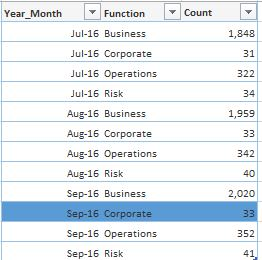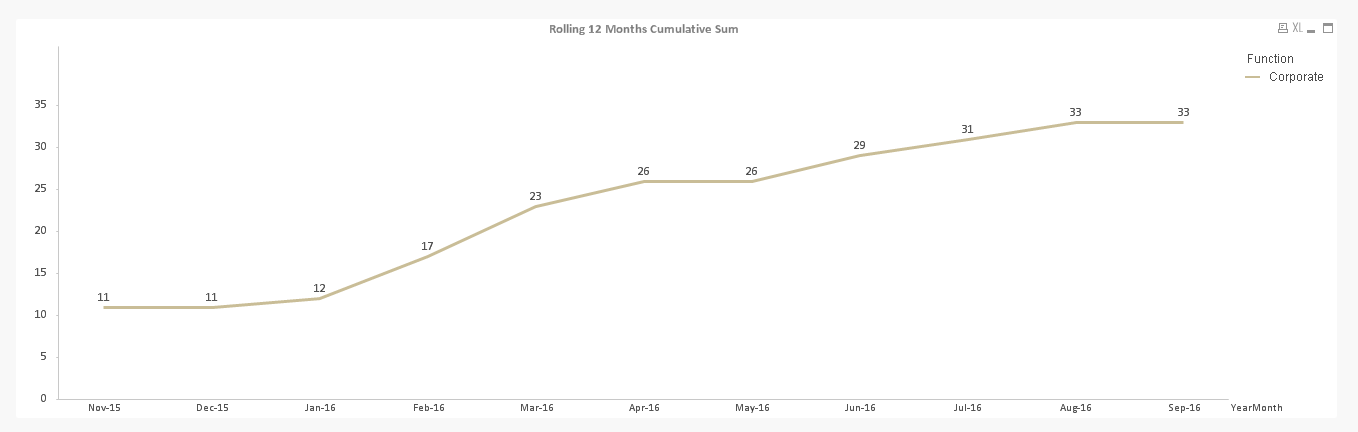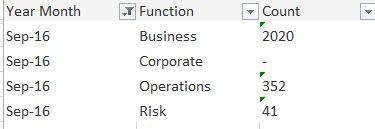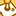# QlikView App Dev

Discussion Board for collaboration related to QlikView App Development.

Announcements
Our next Qlik Insider session will cover new key capabilities. Join us August 11th REGISTER TODAY
cancel
Showing results for
Did you mean:Not applicable

## Rolling 12 months cumulative sum in Line Chart

Hi Everyone,

If current month value of a particular Function is zero ,I want to show Previous month value for Corresponding Function .

Dimensions: -Year_Month and Function

Expressions:

1st Method

=Count({\$<Attr_Flag = {1},Year_Month= {">=\$(=Date(Addmonths(Max(Year_Month),-11),'MMM-YY'))<=\$(=Date(Max(Year_Month),'MMM-YY'))"},Year = ,Month =  >}[Employee Code])

with  Full Accumulation option enabled

2nd Method

Year = ,Month =  >}[Employee Code]),0,Rowno())),Function,Year_Month)

with no accumulation option enabled

I have used above  expressions but still i am not able to get the correct output.

Any suggestions would be greatly appreciated.

Current output:Expected Output:Regards,

P.Divya

1 Solution

Accepted SolutionsMVP

Don't really understand why yours is not working, but this seems to be working:

Alt(Aggr(RangeSum(Above(Alt(Count({\$<Attr_Flag = {1},Year_Month = {">=\$(=Date(Addmonths(Max(Year_Month),-11),'MMM-YY'))<=\$(=Date(Max(Year_Month),'MMM-YY'))"} ,Year = ,Month =  >}[Employee Code]), 0), 0, Rowno())), Function,Year_Month),

Aggr(Above(Aggr(RangeSum(Above(Alt(Count({\$<Attr_Flag = {1},Year_Month = {">=\$(=Date(Addmonths(Max(Year_Month),-11),'MMM-YY'))<=\$(=Date(Max(Year_Month),'MMM-YY'))"} ,Year = ,Month =  >}[Employee Code]), 0), 0, Rowno())), Function,Year_Month)), Function,Year_Month))7 RepliesMVP

It seems like a case of missing dimension. Do you even have Sep-2016 Year-Month for Corporate within your application? Put it another way, is the combination null or is missing? If it is missing, then you probably would need to get this fixed in the script of data source.Not applicable
Author

Hi Sunny ,

Thanks for your response.It is showing null value .I have disabled Suppress Zero values Option.How to get Previous month value for corporate function to Sep-16.I am using the below Expression

Max(Aggr(Rangesum(Above(Count({\$<Attr_Flag = {1},Year_Month= {">=\$(=Date(Addmonths(Max(Year_Month),-11),'MMM-YY'))<=\$(=Date(Max(Year_Month),'MMM-YY'))"},Year = ,Month =  >}[Employee Code]),0,Rowno())),Function,Year_Month))

Regards,

P.DivyaMVP

Would you be able to share a sample to take a look at thisNot applicable
Author

Hi Sunny ,

Please find the attached Sample Application.

Thanks & Regards,

P.DivyaMVP

Don't really understand why yours is not working, but this seems to be working:

Alt(Aggr(RangeSum(Above(Alt(Count({\$<Attr_Flag = {1},Year_Month = {">=\$(=Date(Addmonths(Max(Year_Month),-11),'MMM-YY'))<=\$(=Date(Max(Year_Month),'MMM-YY'))"} ,Year = ,Month =  >}[Employee Code]), 0), 0, Rowno())), Function,Year_Month),

Aggr(Above(Aggr(RangeSum(Above(Alt(Count({\$<Attr_Flag = {1},Year_Month = {">=\$(=Date(Addmonths(Max(Year_Month),-11),'MMM-YY'))<=\$(=Date(Max(Year_Month),'MMM-YY'))"} ,Year = ,Month =  >}[Employee Code]), 0), 0, Rowno())), Function,Year_Month)), Function,Year_Month))Not applicable
Author

Hi Sunny,

Thanks .It is workingRegards,

P.DivyaContributor III

If in bar chart with 2 dimentions, 1st dimention is month, 2nd is customer.

Is it really not possible to show customer value of previous month if this customer does not exist in current month direct in chart function?Community Browser